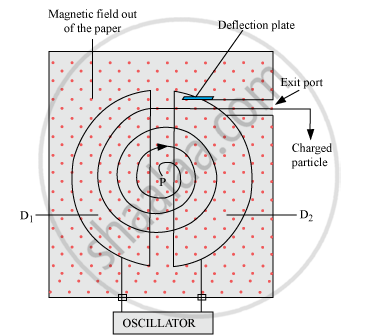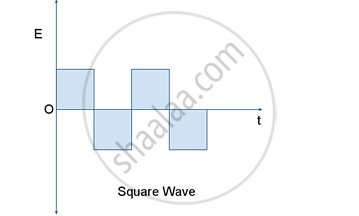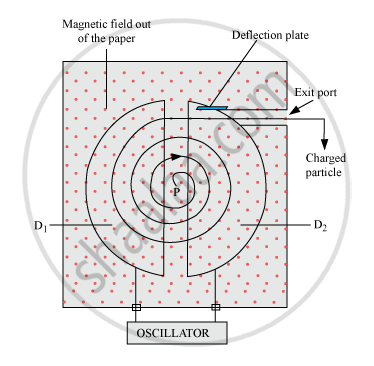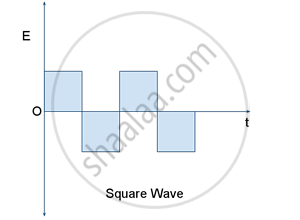Share

# State the underlying principle of a cyclotron. Write briefly how this machine is used to accelerate charged particles to high energies - Physics

#### Questions

State the underlying principle of a cyclotron. Write briefly how this machine is used to accelerate charged particles to high energies

Draw a schematic sketch of a cyclotron. Explain, giving the essential details of its construction, how it is used to accelerate the charged particles.

#### Solution 1

The underlying principle of a cyclotron is that an oscillating electric field can be used to accelerate a charge particle to high energy.

A cyclotron involves the use of an electric field to accelerate charge particles across the gap between the two D-shaped magnetic field regions. The magnetic field is perpendicular to the paths of the charged particles that makes them follow in circular paths within the two Ds. An alternating voltage accelerates the charged particles each time they cross the Ds. The radius of each particle’s path increases with its speed. So, the accelerated particles spiral toward the outer wall of the cyclotron[The Ds are the semi-circular structures (D1 and D2) between which the charges move. The accelerating voltage is maintained across the opposite halves of the Ds.]

Square wave electric fields are used to accelerate the charged particles in a cyclotronThe accelerating electric field reverses just at the time the charge particle finishes its half circle so that it gets accelerated across the gap between the Ds.

The particle gets accelerated again and again, and its velocity increases. Therefore, it attains high kinetic energy

#### Solution 2

It consists of two D-shaped hollow semicircular metal chambers D1 and D2, which are called dees. The two dees are placed horizontally with a small gap separating them. The dees are connected to the source of high frequency electric field. The dees are enclosed in a metal box containing a gas at a low pressure of the order of 10-3 mm mercury. The whole apparatus is placed between two poles of an electromagnet. The magnetic field acts perpendicular to the dees. The positive ions are produced in the gap between the two dees by ionisation of the gas.A cyclotron involves the use of an electric field to accelerate charged particles across the gap between the two D-shaped magnetic field regions. The magnetic field is perpendicular to the paths of the charged particles that makes them follow in circular paths within the two dees. An alternating voltage accelerates the charged particles each time they cross the dees. The radius of each particle’s path increases with its speed. So, the accelerated particles spiral toward the outer wall of the cyclotron

Square wave electric fields are used to accelerate the charged particles in a cyclotron.The accelerating electric field reverses just at the time the charged particle finishes its half circle, so that it gets accelerated across the gap between the dees.

The particle gets accelerated again and again, and its velocity increases. Therefore, it attains high kinetic energy

Is there an error in this question or solution?